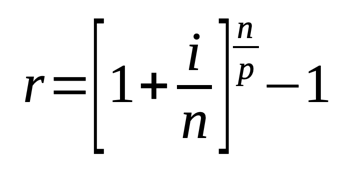# Automobile / Car Loan Calculator

Share:

This calculator is used to calculate the Automobile / Car Loan. You can use this calculator to calculate the periodic payment amount of auto loans along with the upfront payment and the total cost of the automobile.

Enter the required fields and press the Calculate Auto Loan button to get the result.

*
\$
*
Years
Months
*
%
(or)
\$
\$
\$
\$
%
\$
(Registration, Title & Other Fees)
(See your state's sales tax details here)

## About Automobile / Car Loan CalculatorThis calculator is used to calculate the Automobile / Car Loan. You can use this calculator to calculate the periodic payment amount of auto loans along with the upfront payment and the total cost of the automobile.

### Using

1. Enter the Automobile Price (price of the car or automobile).
2. Enter the Loan Term either in years or months.
3. Enter the yearly compound Interest Rate in percentage.
4. Optionally you can select your credit score. Based on the credit score range, the interest rate will be changed. The interest for the credit score range is based on the State of the Automotive Finance Market (Q2 2022) from Experian
6. Enter the Owed on Trade-in value of your old car. You may have to spend more if you have a negative equity on your old car which you are planning to trade-in.
7. Enter the Down Payment amount.
8. Enter the Cash Rebate amount on the new car.
9. Enter the Sales Tax percentage of your state and Other Fees like registration fee, title fee, etc. You can find the approximate sales tax and other fees here.
10. Finally, press the Calculate Auto Loan button. The monthly payment amount, total cost of the automobile, total loan amount, total loan to be paid, sales tax amount, total interest amount, upfront payment amount and effective annual rate are calculated and the result will be displayed.

## Automobile Loan

Automobile loan comes under the category of amortized loan type, in which a fixed payment is paid periodically till it matures.

### Formula To Find Automobile Loan Periodic Payments

This formula is used for calculating repayment of loan amount per period.##### Where:
• A = Repayment of loan amount per period
• P = Loan Amount
• r = Interest Rate per period
• n = Total number of payments or total number of periods

#### Formula To Find Interest Rate per Period##### Where:
• r = Interest rate per period
• i = Nominal annual interest rate
• n = Number of compounding periods / year
• p = Number of payment period / year